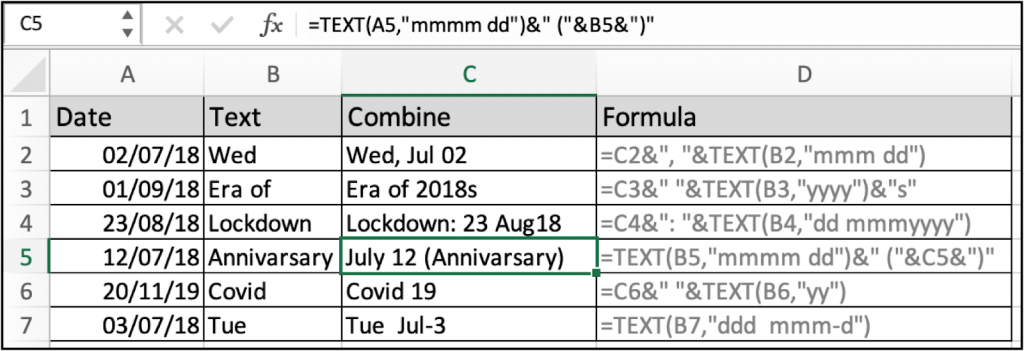# How to Combine Text and Date into a Single Entity in ExcelIn this article, we will learn How to Combine Text and a Formatted Date into a Single Entity in Excel.

Scenario:

In Excel, dates and time values are stored as numbers and are used in numerical calculation by the function. But the problem is the date format and its actual serial. For example, the date 02/07/2018 is stored as the numeric value as 43283. So if we combine a text and a date it would be like 43283, Wed but we need a new format. Let's learn how to convert a date value to text format using text function and combine the two using the functions or operators explained below.

Combine text and date formula in Excel

Text function converts date values (numeric) to date values (text). And then you can use either the CONCATENATE function or the & operator explained below.

CONCATENATE formula excel :

 =CONCATENATE (text, " " , TEXT (date,"format") )

& operator formula :

 =text & " " & TEXT (date,"format")

text : text value to combine

date : date value to combine

format : use the below types to access different date formats. Format type, description and explanation mentioned below in the table

 Type Description Explanation d Day of month d : one or two-digit number without a leading zero (1, 2, 3, ..) dd : two-digit number with a leading zero (like 01, 02, 03, ..) day of week ddd : three letter abbreviation (Mon to Sun) dddd : full name of day of week (Monday to Sunday) m Month m : one or two-digit number without a leading zero (1, 2, ..) mm : two-digit number with a leading zero (01, 02, ..) mmm : abbreviated month (Jan to Dec) mmmm : full name of month (January to December) y Year yy : two-digit number (e.g. 06 meaning 2006 or 16 meaning 2016) yyyy : four digit number (e.g. 2006, 2016) h Hour h : one or two-digit number without a leading zero (1 to 24) hh : two-digit number with a leading zero (01 to 24) m Minute m : one or two-digit number without a leading zero (1 to 60) mm : two-digit number with a leading zero (01 to 60) s Second s : one or two-digit number without a leading zero (1 to 60) ss : two-digit number with a leading zero (01 to 60) AM/PM Time zone Time represented as a 12-hour clock, followed by "AM" or "PM"

Example :

All of these might be confusing to understand. Let's understand how to use the function using an example. Here we have data and we need to add the date value with date values. So here we learn how we can use different text values with date values in excel.

Use the formula:

 =TEXT(A5,"mmmm dd") & " (" & B5 & ")"

Explanation

First text function converts numeric date value 12/07/18 to July 12.

Now & operator joins July 10 with " (" with "Anniversary" with ")".As you can see, the result comes out to be "july 12 (Anniversary)" as text. In the above A5 is reference to the date value and B5 is reference to the text value.

You can also learn the different types of formats to join with text here.

Here are all the observational notes using the formula in Excel
Notes :

1. Excel stores dates and time values as numbers. So it’s recommended to use dates as cell reference or using the DATE function instead of giving direct argument to the function. Check the validation of date values, or else the function returns #VALUE! Error.
2. You can use CONCATENATE function or use & operator to join text in excel.

Hope this article about How to Combine Text and a Formatted Date into a Single Entity in Excel is explanatory. Find more articles on calculating values and related Excel formulas here. If you liked our blogs, share it with your friends on Facebook. And also you can follow us on Twitter and Facebook. We would love to hear from you, do let us know how we can improve, complement or innovate our work and make it better for you. Write to us at info@exceltip.com.

Related Articles :

How to use the WORKDAYS function in Excel : Returns a workday date value after days added to the start_date using the WORKDAYS function in Excel.

Count holidays between dates in Excel : count non working days between the two given dates using the DATEDIF and NETWORKDAYS function in Excel.

SUM price by weekdays in Excel : sum price values corresponding to the number of dates in a particular weekday using the SUMPRODUCT and WEEKDAY function in Excel.

How to Convert date to text in Excel : In this article we learned how to convert text into date, but how do you convert an excel date into text. To convert an excel date into text we have a few techniques.

Extract days between dates ignoring years in Excel : count the days between the two dates using different functions and mathematical operation in Excel

How to Convert date to text in Excel : In this article we learned how to convert text into date, but how do you convert an excel date into text. To convert an excel date into text we have a few techniques.

Popular Articles :